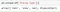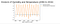# Performing Analysis of Meteorological Data

Hypothesis to be tested : The Influence of Global Warming on temperature and humidity.

We will be performing our analysis on a Weather data. The dataset can be downloaded from Kaggle using the following link :- https://www.kaggle.com/muthuj7/weather-dataset

The dataset has hourly temperature recorded for last 10 years starting from 2006–04–01 00:00:00.000 +0200 to 2016–09–09 23:00:00.000 +0200. It corresponds to Finland, a country in the Northern Europe.

The Null Hypothesis is “Has the Apparent temperature and humidity compared monthly across 10 years of the data indicate an increase due to Global warming.

The hypothesis means we need to find whether the average Apparent temperature for the month of a month say April starting from 2006 to 2016 and the average humidity for the same period have increased or not. This monthly analysis will be done for all 12 months over the 10 year period.

Step 1 : Importing the Dataset

First we will read the dataset named ‘weatherHistory.csv’

`import pandas as pddf = pd.read_csv('weatherHistory.csv')df`

Our dataset contains 96453 rows and 12 columns.

The following columns are present in our weather dataset :

Formatted Date, Summary, Precipitation Type, Temperature ©, Apparent Temperature ©, Humidity, Wind Speed (km/h), Wind Bearing (degrees), Visibility (km), Loud Cover, Pressure (millibars) and Daily Summary.

Step 2 : Data Cleaning

Counting the number of missing values in each column :

`missing_values_count = df.isnull().sum()# get the number of missing data points per columnmissing_values_count`

The column ‘Precip Type’ has 517 missing values.

`pd.unique(df['Precip Type'])`An array with all the unique values in the column ‘Precip Type’ is returned

We are using Pandas’ unique() method to get all types of unique values in the column ‘Precip Type’. As we can see, the output is an array with 3 unique values : ‘rain’, ‘snow’ and nan.

NaN (Not a Number) means missing data. Missing data is labelled NaN. In Pandas, a null value is considered missing and is denoted by NaN.

Drop the rows having missing values :

`df.dropna(how='any', inplace=True)`

This will drop any row with any value missing.

Even if some values are available in a row, it will still get dropped even if a single value is missing.

`df`

Previously, our dataset had 96453 rows and 12 columns.

But since the column ‘Precip Type’ had 517 missing values, so 517 rows are dropped.

So now, our dataset contains 95936 rows and 12 columns.

Step 3 : Working with Date

Displaying column ‘Formatted Date’

`df['Formatted Date']`

Checking the data type of the column ‘Formatted Date’ :

`df['Formatted Date'].dtype# check the data type of our date column`

Here, “O” is the code for “object”.

That means the column ‘Formatted Date’ is of data type ‘object’.

We have to convert the “Formatted Date” column which is in object form to “Datetime” format.

`df['Formatted Date'] = pd.to_datetime(df['Formatted Date'], utc=True)`

Now let us check the data type of the column ‘Formatted Date’ again :

`df['Formatted Date'].dtype`
`df['Formatted Date']`

So, we have converted the “Formatted Date” column into “Datetime” format.

Step 4: Resampling our data from hourly to monthly

Setting Index column using set_index() method :

`df.set_index("Formatted Date", inplace = True)df`

“Formatted Date” column has been made the index column of the dataframe.

Now, we will resample our time series data from hourly daily data into monthly data.

`df_resampled = df.resample('M').mean()df_resampled`

Here, ‘M’ indicates month.

“M” specifies that we have aggregated or resampled our data based on month. The above command will find the mean value of each month (from 2006 to 2016) for all the numerical columns.

There are 8 columns with numerical data.

Step 5 : Performing analysis for testing the given Hypothesis

The hypothesis was to find whether the average Apparent temperature for each month starting from 2006 to 2016 and the average humidity for the same period have increased or not.

So, we will be performing our analysis on these two columns — ‘Apparent Temperature ©’ and ‘Humidity’.

`app_temp = df_resampled['Apparent Temperature (C)']hum = df_resampled['Humidity']`

We have filtered the values in the ‘Apparent Temperature ©’ and ‘Humidity’ columns and saved the outputs to the new dataframes “app_temp” and “hum” respectively.

`import matplotlib.pyplot as plt`

We have imported the pyplot module from matplotlib library.

Matplotlib is a plotting library for creating static, animated, and interactive visualizations in Python.

Pyplot is a Matplotlib module which provides a MATLAB-like interface.

First, let’s plot the data for all the months starting from year 2006 to 2016.

`plt.title('Analysis of Humidity and Temperature (2006 to 2016)', fontsize=25)plt.plot(hum, label="Average Humidity")plt.plot(app_temp, label="Average Apparent Temperature")plt.xlabel("Years (2006 to 2016)", fontsize=13)plt.legend(loc=(1.01,0.8))`Analysing data for all the months starting from year 2006 to 2016.

Now, we have to do monthly analysis for all 12 months over the 10 year period and compare the same month over the 10 year period.

# January :

`df_Jan = df_resampled[df_resampled.index.month==1]hum1 = df_Jan['Humidity']app_temp1 = df_Jan['Apparent Temperature (C)']plt.figure(figsize=(10,5))plt.title('For January (2006 to 2016)', fontsize=25)plt.plot(hum1, label="Average Humidity", marker=".")plt.plot(app_temp1, label="Average Apparent Temperature", marker=".")plt.xlabel("Years (2006 to 2016)", fontsize=13)plt.legend(loc=(1.01,0.8))`

As we can see, the Apparent Temperature had a maximum rise on the year 2007 and again dropped sharply on the year 2010. The Apparent Temperature is varying throughout all the years.

There isn’t any significant change in the Humidity throughtout the years.

# February :

`df_Feb = df_resampled[df_resampled.index.month==2]hum2 = df_Feb['Humidity']app_temp2 = df_Feb['Apparent Temperature (C)']plt.figure(figsize=(10,5))plt.title('For February (2006 to 2016)', fontsize=25)plt.plot(hum2, label="Average Humidity", marker=".", linestyle="dashed")plt.plot(app_temp2, label="Average Apparent Temperature", marker=".")plt.xlabel("Years (2006 to 2016)", fontsize=13)plt.legend(loc=(1.01,0.8))`

# March :

`df_March = df_resampled[df_resampled.index.month==3]hum3 = df_March['Humidity']app_temp3 = df_March['Apparent Temperature (C)']plt.figure(figsize=(10,5))plt.title('For March (2006 to 2016)', fontsize=25)plt.plot(hum3, label="Average Humidity", marker=".")plt.plot(app_temp3, label="Average Apparent Temperature", marker=".")plt.xlabel("Years (2006 to 2016)", fontsize=13)plt.legend(loc=(1.01,0.8))`

# April :

`df_April = df_resampled[df_resampled.index.month==4]hum4 = df_April['Humidity']app_temp4 = df_April['Apparent Temperature (C)']plt.figure(figsize=(10,5))plt.title('For April (2006 to 2016)', fontsize=25)plt.plot(hum4, label="Average Humidity", marker=".")plt.plot(app_temp4, label="Average Apparent Temperature", marker=".")plt.xlabel("Years (2006 to 2016)", fontsize=13)plt.legend(loc=(1.01,0.8))`

# May :

`df_May = df_resampled[df_resampled.index.month==5]hum5 = df_May['Humidity']app_temp5 = df_May['Apparent Temperature (C)']plt.figure(figsize=(10,5))plt.title('For May (2006 to 2016)', fontsize=25)plt.plot(hum5, label="Average Humidity", marker=".")plt.plot(app_temp5, label="Average Apparent Temperature", marker=".")plt.xlabel("Years (2006 to 2016)", fontsize=13)plt.legend(loc=(1.01,0.8))`

# June :

`df_June = df_resampled[df_resampled.index.month==6]hum6 = df_June['Humidity']app_temp6 = df_June['Apparent Temperature (C)']plt.figure(figsize=(10,5))plt.title('For June (2006 to 2016)', fontsize=25)plt.plot(hum6, label="Average Humidity", marker=".")plt.plot(app_temp6, label="Average Apparent Temperature", marker=".")plt.xlabel("Years (2006 to 2016)", fontsize=13)plt.legend(loc=(1.01,0.8))`

# July :

`df_July = df_resampled[df_resampled.index.month==7]hum7 = df_July['Humidity']app_temp7 = df_July['Apparent Temperature (C)']plt.figure(figsize=(10,5))plt.title('For July (2006 to 2016)', fontsize=25)plt.plot(hum7, label="Average Humidity", marker=".", color="green")plt.plot(app_temp7, label="Average Apparent Temperature", marker=".", color="red")plt.xlabel("Years (2006 to 2016)", fontsize=13)plt.legend(loc=(1.01,0.8))`

# August :

`df_Aug = df_resampled[df_resampled.index.month==8]hum8 = df_Aug['Humidity']app_temp8 = df_Aug['Apparent Temperature (C)']plt.figure(figsize=(10,5))plt.title('For August (2006 to 2016)', fontsize=25)plt.plot(hum8, label="Average Humidity", marker=".")plt.plot(app_temp8, label="Average Apparent Temperature", marker=".")plt.xlabel("Years (2006 to 2016)", fontsize=13)plt.legend(loc=(1.01,0.8))`

# September :

`df_Sep = df_resampled[df_resampled.index.month==9]hum9 = df_Sep['Humidity']app_temp9 = df_Sep['Apparent Temperature (C)']plt.figure(figsize=(10,5))plt.title('For September (2006 to 2016)', fontsize=25)plt.plot(hum9, label="Average Humidity", marker=".")plt.plot(app_temp9, label="Average Apparent Temperature", marker=".")plt.xlabel("Years (2006 to 2016)", fontsize=13)plt.legend(loc=(1.01,0.8))`

# October :

`df_Oct = df_resampled[df_resampled.index.month==10]hum10 = df_Oct['Humidity']app_temp10 = df_Oct['Apparent Temperature (C)']plt.figure(figsize=(10,5))plt.title('For October (2006 to 2016)', fontsize=25)plt.plot(hum10, label="Average Humidity", marker=".")plt.plot(app_temp10, label="Average Apparent Temperature", marker=".")plt.xlabel("Years (2006 to 2016)", fontsize=13)plt.legend(loc=(1.01,0.8))`

# November :

`df_Nov = df_resampled[df_resampled.index.month==11]hum11 = df_Nov['Humidity']app_temp11 = df_Nov['Apparent Temperature (C)']plt.figure(figsize=(10,5))plt.title('For November (2006 to 2016)', fontsize=25)plt.plot(hum11, label="Average Humidity", marker=".")plt.plot(app_temp11, label="Average Apparent Temperature", marker=".")plt.xlabel("Years (2006 to 2016)", fontsize=13)plt.legend(loc=(1.01,0.8))`

# December :

`df_Dec = df_resampled[df_resampled.index.month==12]hum12 = df_Dec['Humidity']app_temp12 = df_Dec['Apparent Temperature (C)']plt.figure(figsize=(10,5))plt.title('For December (2006 to 2016)', fontsize=25)plt.plot(hum12, label="Average Humidity", marker=".")plt.plot(app_temp12, label="Average Apparent Temperature", marker=".")plt.xlabel("Years (2006 to 2016)", fontsize=13)plt.legend(loc=(1.01,0.8))`

Conclusion :

After performing analysis on the weather data, we can see from the monthly analysis that the Apparent Temperature for each month has seen significant variations throughtout the period of 10 years.

But the month analysis of Humidity shows that there isn’t any significant changes for all 12 months over the 10 year period.